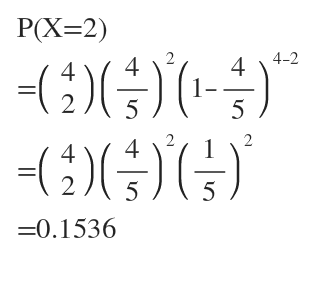# Suppose a coin with Pr[H]=4/5Pr[H]=4/5 is flipped 4 times. Answer the following questions:1. What is the probability exactly 2 heads were flipped?  2. What is the probability that heads then tails then heads then tails was flipped?

Question
22 views

Suppose a coin with Pr[H]=4/5Pr[H]=4/5 is flipped 4 times. Answer the following questions:

1. What is the probability exactly 2 heads were flipped?

2. What is the probability that heads then tails then heads then tails was flipped?

check_circle

Step 1

Given Pr(H)= 4/5

Number of trials = 4

X= number of times head comes

Each trial is independent so, it is Bernoulli trial.

1)

We have to find P(X=2)...

### Want to see the full answer?

See Solution

#### Want to see this answer and more?

Solutions are written by subject experts who are available 24/7. Questions are typically answered within 1 hour.*

See Solution
*Response times may vary by subject and question.
Tagged in